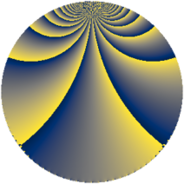# Properties

 Label 1368.3.bdLevel $1368$ Weight $3$ Character orbit 1368.bd Rep. character $\chi_{1368}(107,\cdot)$ Character field $\Q(\zeta_{6})$ Dimension $320$ Sturm bound $720$

# Related objects

## Defining parameters

 Level: $$N$$ $$=$$ $$1368 = 2^{3} \cdot 3^{2} \cdot 19$$ Weight: $$k$$ $$=$$ $$3$$ Character orbit: $$[\chi]$$ $$=$$ 1368.bd (of order $$6$$ and degree $$2$$) Character conductor: $$\operatorname{cond}(\chi)$$ $$=$$ $$456$$ Character field: $$\Q(\zeta_{6})$$ Sturm bound: $$720$$

## Dimensions

The following table gives the dimensions of various subspaces of $$M_{3}(1368, [\chi])$$.

Total New Old
Modular forms 976 320 656
Cusp forms 944 320 624
Eisenstein series 32 0 32

## Trace form

 $$320q + 4q^{4} + O(q^{10})$$ $$320q + 4q^{4} - 60q^{10} - 28q^{16} + 64q^{19} - 800q^{25} - 12q^{34} - 2176q^{49} - 240q^{52} - 128q^{58} - 368q^{64} - 864q^{70} + 160q^{73} - 692q^{76} - 116q^{82} + O(q^{100})$$

## Decomposition of $$S_{3}^{\mathrm{new}}(1368, [\chi])$$ into newform subspaces

The newforms in this space have not yet been added to the LMFDB.

## Decomposition of $$S_{3}^{\mathrm{old}}(1368, [\chi])$$ into lower level spaces

$$S_{3}^{\mathrm{old}}(1368, [\chi]) \cong$$ $$S_{3}^{\mathrm{new}}(456, [\chi])$$$$^{\oplus 2}$$